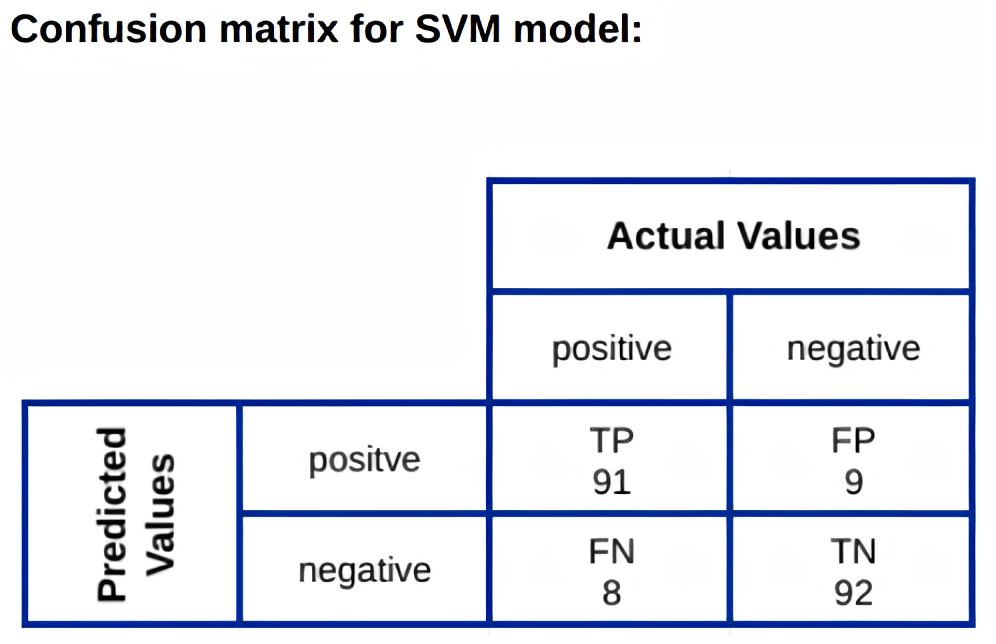Sentiment analysis is a sub field of Natural Language Processing (NLP) that identifies and extracts emotions expressed in given texts. It is a machine learning tool that understands the context and determines the polarity of text, whether it is positive, neutral, or negative.

This article will discuss what sentiment analysis is, where it is being used, and how to use a pre-trained model to analyze sentiments from texts.

We will also explore the approach on how Machine Learning models are used to build sentiment analytic tools.

### Use cases of sentiment analysis:

• Brand Monitoring
• Customers Feedback
• Product Analytics
• Monitoring Market Research
• Analyzing Movie Reviews

There are various pre-trained sentiment analysis tools available in Natural Language Processing (NLP) libraries. Such as NLTK’s Vader sentiment analysis tool, TextBlob, Flair sentiment classifier based on LSTM neural network, etc.

### Part 1- Sentiment analysis using a pre-trained model (TextBlob)

TextBlob is a python library for Natural Language Processing (NLP). It helps you perform complex analysis and operations on textual data.

#### Steps to apply the TextBlob model to achieve sentiments are given here:

Before applying Textblob, basic text cleaning should be done. You can check NLTK or Spacy libraries for various text cleaning methods.

from textblob import TextBlob

def sentimental(text: str) -> str:

sentiment = None

if text:

text = ‘ ‘.join(text.split()).strip() # removing empty strings

blob = TextBlob(text)

if blob.sentiment.polarity > 0:

sentiment = ‘Positive’

if blob.sentiment.polarity < 0:

sentiment = ‘Negative’

if blob.sentiment.polarity == 0:

sentiment = ‘Neutral’

return sentiment

#### Output Result:

sentimental(“This is what a true masterpiece looks like. Thrillingly played with a flawless ensemble cast.”)

Out: ‘Positive

TextBlob returns the ‘polarity’ of a sentence. Polarity lies between [-1,1].

-1 defines Negative, 0 defines Neutral, and 1 defines Positive.

### Part 2 – Train a Machine Learning Model for sentiment analysis

In this part, we will be using a Supervised Machine Learning model called Support Vector Machines (SVM) to train the model.

#### Data Gathering:

Here we will choose sentiment polarity datasets 2.0 which is a classified movie dataset with labels, and transformed into CSVs.

Data is divided into “trainData” and “testData”. The dataset contains “Content” and “Label” columns.#### Data Vectorization

Before feeding our model with data, we need to extract features from our textual dataset, basically converting the text data into vectors. TF-IDF is one of many methods to extract features from text documents. TF-IDF stands for ‘Term Frequency – Inverse Document Frequency.

from sklearn.feature_extraction.text import TfidfVectorizer

# Create feature vectors

vectorizer = TfidfVectorizer(min_df = 5,

max_df = 0.8,

sublinear_tf = True,

use_idf = True)

train_vectors = vectorizer.fit_transform(trainData[‘Content’])

test_vectors = vectorizer.transform(testData[‘Content’])

#### Model Building

After generating vectors for both train and test input sets, we can now feed the SVC model with this data and train it.

# importing libraries

from sklearn import svm

from sklearn.metrics import classification_report

# Initialising SVM classifier with linear kernel

svm_classifier = svm.SVC(kernel=’linear’)

# training the model with the train data

svm_classifier.fit(train_vectors, trainData[‘Label’])

# testing the model in test data content

predicted_result = svm_classifier.predict(test_vectors)

# results

report = classification_report(testData[‘Label’], predicted_result, output_dict=True)

print(‘Model accuracy: ‘, report[‘accuracy’])

#### Model Results and Statistics:

Model accuracy:  0.915

Model accuracy shows the ratio of the number of correctly predicted classes to the total number of input samples. Accuracy is one of many metrics used for evaluating classification problems.

Here the accuracy is 0.915, which shows that the model has learned the data quite well as the range of accuracy is calculated between 0 to 1.

#### Testing the Model to Predict on Movie Reviews:

svm_classifier.predict(vectorizer.transform(“This is what a true masterpiece looks like. Thrillingly played with a flawless ensemble cast.”))

Out: ‘PositiveClassification accuracy alone can be misleading if you have an unequal number of observations. A confusion matrix can give you a better idea of what our model is predicting correctly.

Here we have taken 200 test samples and as shown in the matrix above, we got 9 False positives, which means it has falsely predicted the negative as positive. There were also 8 False negatives, where it has falsely predicted the positive as negative.

To reduce these errors we can train the model with a larger dataset.### Conclusion

In this article, we have mentioned the TextBlob (pre-trained) Python package and SVM (Machine Learning) model to determine sentiment analysis. But the field of sentiment analysis is an exciting research direction due to a large number of real-world applications where discovering people’s opinions is important in better decision-making.

Although detecting sentiment using NLP is surprisingly a difficult task, such as when we face sentences that are put in sarcastic ways. These types of textual context can mislead NLP-based model predictions. We can even see that both the model prediction results are not the same for all samples. Here the TextBlob model performs and predicts better with ‘neutral’ tagging of articles. This is because TextBlob is using more data to train the model and has neutral tagged data in the training set.

To overcome such difficult tasks, we can use deep learning models like LSTM, RNN, etc. We can even make use of transformer-based models like GPT-3 and T5 from google for sentiment analysis.